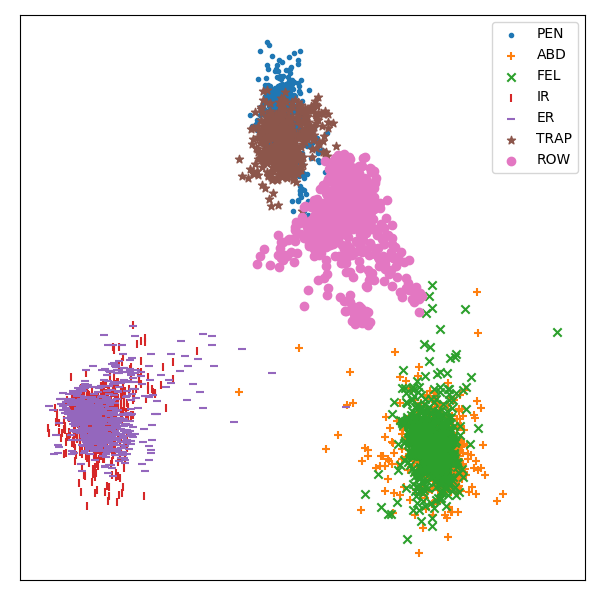# Linear Discriminant Analysis¶

This example demonstrates how the pipeline can be used to perform transformation of time series data, such as linear discriminant analysis for visualization purposesOut:

```/home/circleci/miniconda/envs/testenv/lib/python3.8/site-packages/sklearn/utils/validation.py:67: FutureWarning: Pass memory=None as keyword args. From version 0.25 passing these as positional arguments will result in an error
warnings.warn("Pass {} as keyword args. From version 0.25 "
/home/circleci/miniconda/envs/testenv/lib/python3.8/site-packages/seglearn/transform.py:237: VisibleDeprecationWarning: Creating an ndarray from ragged nested sequences (which is a list-or-tuple of lists-or-tuples-or ndarrays with different lengths or shapes) is deprecated. If you meant to do this, you must specify 'dtype=object' when creating the ndarray
Xt = np.array([sliding_tensor(Xt[i], self.width, self._step, self.order)
```

```# Author: David Burns

import matplotlib.pyplot as plt
import numpy as np
from sklearn.discriminant_analysis import LinearDiscriminantAnalysis

import seglearn as sgl

def plot_embedding(emb, y, y_labels):
# plot a 2D feature map embedding
x_min, x_max = np.min(emb, 0), np.max(emb, 0)
emb = (emb - x_min) / (x_max - x_min)

NC = len(y_labels)
markers = ['.', '+', 'x', '|', '_', '*', 'o']

fig = plt.figure()
fig.set_size_inches(6, 6)

for c in range(NC):
i = y == c
plt.scatter(emb[i, 0], emb[i, 1], marker=markers[c], label=y_labels[c])

plt.xticks([]), plt.yticks([])
plt.legend()
plt.tight_layout()

X = data['X']
y = data['y']

# create a pipeline for LDA transformation of the feature representation
clf = sgl.Pype([('segment', sgl.Segment()),
('ftr', sgl.FeatureRep()),
('lda', LinearDiscriminantAnalysis(n_components=2))])

X2, y2 = clf.fit_transform(X, y)
plot_embedding(X2, y2.astype(int), data['y_labels'])
plt.show()
```

Total running time of the script: ( 0 minutes 0.756 seconds)

Gallery generated by Sphinx-Gallery